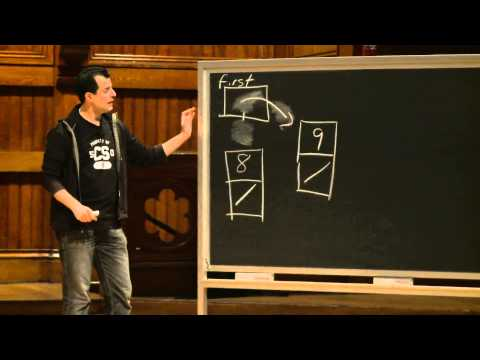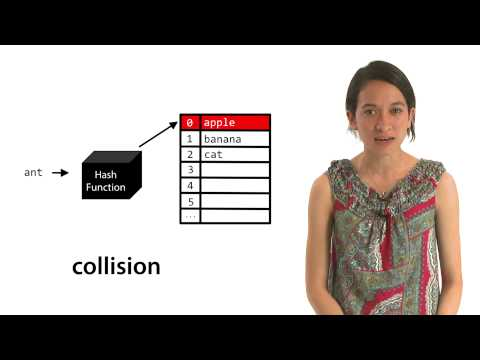# Hash Tables

## Notes

Hash tables are used when speedy insertion, deletion, and lookup is the priority. In fact, for an ideally tuned hash table, insertion, deletion, and lookup can be accomplished in constant time.

A hash table is an array associated with a function (the hash function).

This function maps keys to array indices. For example, in this slide we see that the hash function has mapped the key 'banana' to index 1.

But what's going on under the hood?

The hash function takes a key as input and computes an array index from the intrinsic properties of that key.

You’d initially use the hash function to determine where in the hash table to store a given key. Later, you’d use the same hash function to determine where in the hash table to search for a given key. For this reason, it’s crucial that a hash function behaves consistently and outputs the same index for identical inputs.

Keep in mind that hash tables can be used to store data of all types, but for now, let’s consider a very simple hash function for strings.

This hash function uses the first letter of a string to determine a hash table index for that string, so words that start with the letter 'a' are assigned to index 0, 'b' to index 1, and so on.

Note that this hash function returns hash % SIZE, where SIZE is the size of the hash table. Modding by the size of the hash table is a good way to avoid indexing into a hash table slot that does not exist.

Time to throw a wrench into things. What if we want to store the word berry into the table as well?

Berry hashes to index 1, just as banana did. This is an example of a collision, the result of two keys hashing to the same index.

Even if your hash table is larger than your dataset and you’ve chosen a good hash function, you need a plan for dealing with collisions if and when they arise. Two common methods of dealing with collisions are linear probing and separate chaining.

With linear probing, if a key hashes to the same index as a previously stored key, it is assigned the next available slot in the table.

So banana is stored at index 1, and berry is stored at index 3.

As you can surmise even from this simple example, once a collision occurs, you’ve significantly increased chances that another collision will occur in the same area. This is called clustering, and it’s a serious drawback to linear probing.

Worst case insertion, deletion, and lookup times have devolved to O(n), as the next available slot could potentially have been the last slot in the table.

In the separate chaining model, the hash table is actually an array of pointers to linked lists.

When a collision occurs, the key can be inserted in constant time at the head of the appropriate linked list.

What happens now when we search for banana in the hash table? We must traverse the entire linked list at index 1. The worst case lookup time for a hash table that uses separate chaining (assuming a uniform hash function) is therefore O(n/m), where m is the size of the hash table.

Since m is a constant, O(n/m) is theoretically equivalent to O(n). In the real world, however, O(n/m) is a big improvement over O(n)!

A good hash function will maximize this real world improvement.## Slides ( / )## Hash Function

Prerequisites:

Write a hash function for strings that sums the ASCII values of the argument `key`'s characters. Don’t forget to take into account the size of your hash table!

In the default code, we’ve provided functionality to get a string from the user and print out its hash value (according to your hash function)! Don’t worry about invalid inputs.

``````jharvard@run.cs50.net (~): ./a.out
Hash table size: 100
Key: puppy
The string 'puppy' is mapped to index 14.``````

Try out some pseudocode here!
```#include <cs50.h>
#include <ctype.h>
#include <stdio.h>

int hash_function(char* key, int size)
{
// TODO
}

int main(int argc, char* argv[])
{
// get hash table size
printf("Hash table size: ");
int size = GetInt();

// get key
printf("Key: ");
char* key = GetString();

// calculate and print index
printf("The string '%s' is mapped to index %i\n", key,
hash_function(key, size));

return 0;
}
```

## Videos#### Wednesday, Week 6

An introduction to data structures#### Lauren's Hash Tables Short

Lauren gives a general overview